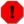# 3. The Solution

## 3.1. extern "C"

C++ has a special keyword to declare a function with C bindings: extern "C". A function declared as extern "C" uses the function name as symbol name, just as a C function. For that reason, only non-member functions can be declared as extern "C", and they cannot be overloaded.

Although there are severe limitations, extern "C" functions are very useful because they can be dynamically loaded using dlopen just like a C function.

This does not mean that functions qualified as extern "C" cannot contain C++ code. Such a function is a full-featured C++ function which can use C++ features and take any type of argument.

In C++ functions are loaded just like in C, with dlsym. The functions you want to load must be qualified as extern "C" to avoid the symbol name being mangled.

main.cpp:

 ```#include #include int main() { using std::cout; using std::cerr; cout << "C++ dlopen demo\n\n"; // open the library cout << "Opening hello.so...\n"; void* handle = dlopen("./hello.so", RTLD_LAZY); if (!handle) { cerr << "Cannot open library: " << dlerror() << '\n'; return 1; } // load the symbol cout << "Loading symbol hello...\n"; typedef void (*hello_t)(); // reset errors dlerror(); hello_t hello = (hello_t) dlsym(handle, "hello"); const char *dlsym_error = dlerror(); if (dlsym_error) { cerr << "Cannot load symbol 'hello': " << dlsym_error << '\n'; dlclose(handle); return 1; } // use it to do the calculation cout << "Calling hello...\n"; hello(); // close the library cout << "Closing library...\n"; dlclose(handle); }```

hello.cpp:

 ```#include extern "C" void hello() { std::cout << "hello" << '\n'; } ```

The function hello is defined in hello.cppas extern "C"; it is loaded in main.cpp with the dlsym call. The function must be qualified as extern "C" because otherwise we wouldn't know its symbol name.There are two different forms of the extern "C" declaration: extern "C" as used above, and extern "C" { … } with the declarations between the braces. The first (inline) form is a declaration with extern linkage and with C language linkage; the second only affects language linkage. The following two declarations are thus equivalent:

 ```extern "C" int foo; extern "C" void bar(); ```

and

 ```extern "C" { extern int foo; extern void bar(); }```

As there is no difference between an extern and a non-extern function declaration, this is no problem as long as you are not declaring any variables. If you declare variables, keep in mind that

 `extern "C" int foo;`

and

 ```extern "C" { int foo; }```

are not the same thing.

For further clarifications, refer to [ISO14882], 7.5, with special attention to paragraph 7, or to [STR2000], paragraph 9.2.4.

Before doing fancy things with extern variables, peruse the documents listed in the see also section.

Loading classes is a bit more difficult because we need an instance of a class, not just a pointer to a function.

We cannot create the instance of the class using new because the class is not defined in the executable, and because (under some circumstances) we don't even know its name.

The solution is achieved through polymorphism. We define a base, interface class with virtual members in the executable, and a derived, implementation class in the module. Generally the interface class is abstract (a class is abstract if it has pure virtual functions).

As dynamic loading of classes is generally used for plug-ins — which must expose a clearly defined interface — we would have had to define an interface and derived implementation classes anyway.

Next, while still in the module, we define two additional helper functions, known as class factory functions. One of these functions creates an instance of the class and returns a pointer to it. The other function takes a pointer to a class created by the factory and destroys it. These two functions are qualified as extern "C".

To use the class from the module, load the two factory functions using dlsym just as we loaded the the hello function; then, we can create and destroy as many instances as we wish.

Here we use a generic polygon class as interface and the derived class triangle as implementation.

main.cpp:

 ```#include "polygon.hpp" #include #include int main() { using std::cout; using std::cerr; // load the triangle library void* triangle = dlopen("./triangle.so", RTLD_LAZY); if (!triangle) { cerr << "Cannot load library: " << dlerror() << '\n'; return 1; } // reset errors dlerror(); // load the symbols create_t* create_triangle = (create_t*) dlsym(triangle, "create"); const char* dlsym_error = dlerror(); if (dlsym_error) { cerr << "Cannot load symbol create: " << dlsym_error << '\n'; return 1; } destroy_t* destroy_triangle = (destroy_t*) dlsym(triangle, "destroy"); dlsym_error = dlerror(); if (dlsym_error) { cerr << "Cannot load symbol destroy: " << dlsym_error << '\n'; return 1; } // create an instance of the class polygon* poly = create_triangle(); // use the class poly->set_side_length(7); cout << "The area is: " << poly->area() << '\n'; // destroy the class destroy_triangle(poly); // unload the triangle library dlclose(triangle); }```

polygon.hpp:

 ```#ifndef POLYGON_HPP #define POLYGON_HPP class polygon { protected: double side_length_; public: polygon() : side_length_(0) {} virtual ~polygon() {} void set_side_length(double side_length) { side_length_ = side_length; } virtual double area() const = 0; }; // the types of the class factories typedef polygon* create_t(); typedef void destroy_t(polygon*); #endif```

triangle.cpp:

 ```#include "polygon.hpp" #include class triangle : public polygon { public: virtual double area() const { return side_length_ * side_length_ * sqrt(3) / 2; } }; // the class factories extern "C" polygon* create() { return new triangle; } extern "C" void destroy(polygon* p) { delete p; } ```